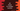# C# program to check if a number is prime or not## C# program to check if a user input number is prime:

In this post, we will learn how to check if a user input number is prime or not. It will take one number as input, check it if it is prime or not and print this result.

### C# program:

A number is called a prime number if it has only by itself and 1. For example, 2,3,5,7,11 are prime numbers but 98 is not.

Below is the complete program:

``````using System;

public class Program
{
private static bool isPrime(int num){
for(int i = 2; i<= num/2; i++){
if(num % i == 0){
return false;
}
}
return true;
}

public static void Main()
{
int num;
Console.WriteLine("Enter the number to check : ");

if(isPrime(num)){
Console.WriteLine("{0} is a prime number",num);
} else {
Console.WriteLine("{0} is not a prime number",num);
}
}
}``````

Here,

• isPrime is used to check if a given number is prime or not. It returns true if it is prime, else false. We can check up to number/2 if any one can divide the number or not. It makes the for loop smaller.
• It is asking the user to enter a number to check. Using ReadLine(), it reads that number and stored it in num.
• Using isPrime, it checks if it is prime or not.
• Based on the return value of isPrime, it prints one message.

### Sample output:

It will print output as like below:

``````Enter the number to check :
98
98 is not a prime number

Enter the number to check :
97
97 is a prime number``````